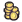Whenever a new a game is started, many settings can be tweaked to adjust the game to your preferences. Some of them are covered below.

# Starting units

"Starting units" defines the strength of the units each player will start with on turn 1. In any case, each player will receive his racial unit.
The AI players receive additional starting units at higher difficulties (Lord and higher).

 Starting unit Weak Normal Strong Battle Value* 160 350 640 1340 Maximum number of units (+ 1 racial ) 3+1 5+1 5+1 12+1

*: the value is calculated through this formula:
([value of the unit]+[[[File:Mana_Text.png|Mana|link=Resources#Mana]] value of the unit]*2)*0.9 (so you can get slightly more units costing onlythan what the value of 160 for Weak starting unit would let you expect for instance)
The value of units is divided by a factor to represent their relative weakness (so you get more units than normal units at equivalent Starting unit strength): 1.3 for Weak and Normal Starting unit, and 1.1 for Strong and Battle Starting unit.